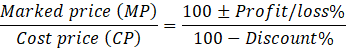Question of The Day01-06-2020

# A shopkeeper gives a successive discount of 30% and 20% on a laptop’s marked price but he sells the laptop such that he gets an effective profit of 10% on the cost price. What is the marked price of laptop if the cost price of laptop is ₹56000?

Correct Answer : d ) ₹ 1,10,000

Explanation :

Quick Approach

We know, for any two successive discounts m and n the effective discount is given as

= $$m+n-{mn \over 100}$$

$$Effective discount will be=30+20-{30×20 \over 100}$$

= 50 – 6 = 44%

Now,$${MP \over 56000}={100+10 \over 100-44}$$

$$MP=56000* {110 \over 56}=₹ 1,10,000$$

Hence, (d) is the correct answer.

Basic Approach

We know,

Selling Price (SP) = Cost Price (CP) + gain% on CP

As, shopkeeper gains 10% on the cost price (C.P.) so,

SP = 56000 + 10% of 56000

= Rs. 61600

For any two successive discounts m and n the effective discount is given as

= $$m+n-{mn \over 100}$$

$$Effective discount will be=30+20-{30×20 \over 100}$$

= 50 – 6 = 44%

Now,

According to the question,

Marked price (MP) = Selling Price (SP) + discount%

MP = 61600 + 44% of MP

$$MP =61600+{44 \over 100} \ of MP$$

0.56 of M.P. = 61600

MP = Rs. 1,10,000

Hence, (d) is the correct answer.

Such type of question is asked in various government exams like SSC CGL, SSC MTS, SSC CPO, SSC CHSL, RRB JE, RRB NTPC, RRB GROUP D, RRB OFFICER SCALE-I, IBPS PO, IBPS SO, RRB Office Assistant, IBPS Clerk, RBI Assistant, IBPS RRB OFFICER SCALE 2&3, UPSC CDS etc.

Read Daily Current Affairs, Banking Awareness, Hindi Current Affairs, Word of the Day, and attempt free mock tests at PendulumEdu and boost your preparation for the actual exam.0